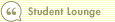Course 403: Introduction to Discounted Cash Flow
The Perpetuity ValueIn this course1 Introduction 2 Estimating Future Cash Flow 3 Discounting and Discount Rates 4 Cost of Capital 5 Two Types of Capital, Two Costs 6 The Perpetuity Value 7 The Bottom LineThe last piece of the puzzle is the perpetuity value. This figure is necessary because it's not feasible to project a company's future cash flows out to infinity, year by year. At some point, we have to stop, even if we believe the company will continue generating profits for a long time. We can solve this problem by estimating a company's future cash flows for a certain period--say five or 10 years--and then estimating the value of all cash flows after that in one lump sum. This lump sum is the perpetuity value.

A company's cost of capital also plays an important part in calculating the perpetuity value. The most common way to do this is to take the last cash flow estimated, increase it by the rate at which you expect cash flows to grow over the long term, and divide the result by the cost of capital minus the estimated growth rate.

Perpetuity Value =
( CFn x (1+ g) ) / R - g

CFn = Cash Flow in the Last Individual Year Estimated
g = Long-Term Growth Rate
R = Discount Rate, or Cost of Capital

To better understand the perpetuity value, suppose we're using a five-year DCF model for a company with a 9% cost of capital. We estimate that the company's free cash flow in Year 5 will be \$100 million, and that its cash flow will grow at 5% after that. The perpetuity value will equal:

( 100 million x (1 + .05) ) / (.09 -.05) = \$2.625 billion

Remember, the perpetuity value is calculated as of five years from now. To find out what the value is today, we have to discount the calculated value using the formula we learned earlier:

Present Value of Perpetuity Value =
\$2.625 billion / (1 + .09)^5 = \$1.706 billion

Once we've found the present value of the perpetuity, we simply add this number to the present value of the cash flows we estimated in Years 1 through 5 to determine the fair value, or intrinsic value, of the company. In the next lesson, we'll walk through a sample DCF model that will help you put this into practice.

Next: The Bottom Line >>Search Print Lesson  | FeedbackDel.icio.us |digg itLearn how to invest like a pro with Morningstar’s Investment Workbooks (John Wiley & Sons, 2004, 2005), available at online bookstores.© Copyright 2015 Morningstar, Inc. All rights reserved. Please read our Privacy Policy. If you have questions or comments please contact Morningstar.